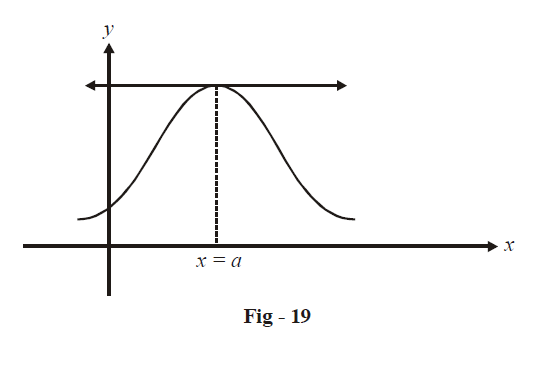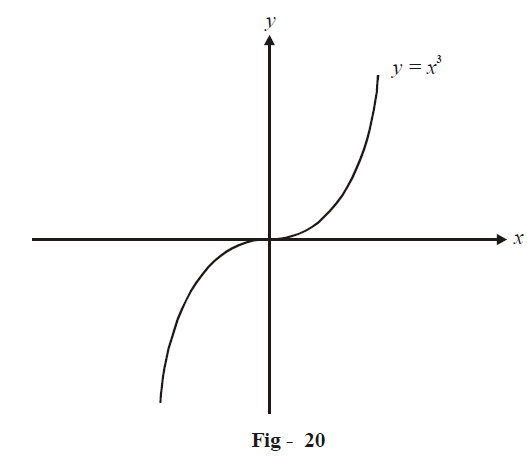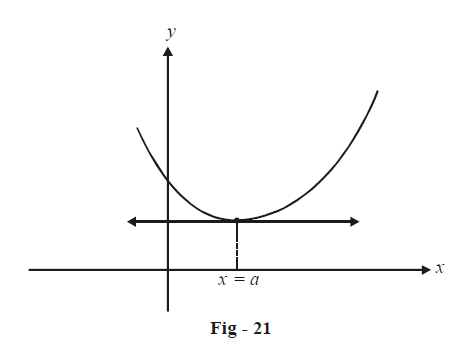# Finding Maxima and Minima Points

Go back to  'Applications of Derivatives'

HOW TO EVALUATE EXTREMUM POINTS ?

Consider a function $$f\left( x \right)$$ that attains a local maximum at $$x=a$$ as shown in the figure below:It is obvious that the tangent drawn to the curve at $$x=a$$ must have 0 slope, i,e, $$f'\left( a \right)=0.$$

This is therefore a necessary condition; however, it is not sufficient.

Consider $$f\left( x \right) = {x^3}.$$Observe that even though $${\left. {f'\left( 0 \right) = 3{x^2}} \right|_{x = 0}} = 0,x = 0$$   is not an extremum point. What is then, the difference between this and the function discussed previously in Fig-19?

In the previous function, observe that f '(x) is positive for x < a and negative for x > a (we only need to focus on the neighbourhood of $$x=a$$ ) i.e, f '(x) changes sign from positive to negative as x crosses a.

What would have happened had $$x=a$$ been a local minimum point?We now see that f '(x) changes sign from negative to positive as x crosses a.

However, for $$f(x){\rm{ }} = {x^3},$$ observe that f '(x) does not change sign as x crosses 0; f '(x) > 0 whether x < 0 or x > 0.

This distinction therefore leads us to our sufficient condition.

x = a is a local maximum for f(x) if

\begin{align}& \text{ }\!\!~\!\!\text{ } {f}'(a)=0 \\ \text{ }\!\!~\!\!\text{ } & {f}'(x)\ \text{changes}\ \text{from}\ \left( +\text{ve} \right)\to \left( -\text{ve} \right)\ \text{as}\ x\ \text{crosses}\ a\ (\text{from}\ \text{left}\ \text{to}\ \text{right})~ \\\end{align}

x = a is a local minimum for f(x) if

\begin{align}& \text{ }\!\!~\!\!\text{ } {f}'(a)=0 \\ \text{ }\!\!~\!\!\text{ } & {f}'(x)\ \text{changes}\ \text{from }\left( -\text{ve} \right)\to \left( \text{+ve} \right)\text{ as}\ x\ \text{crosses}\ a. \\ \end{align}

x = a is not an extremum point for f(x) if

\begin{align}&f'\left( a \right)\text{ }=\text{ }0\\&\text{but}\,f'\left( x \right)\text{does not change sign as}\,x\,\text{crosses}\,a. \end{align}

These straight forward criteria constitute what is known as the First Derivative Test.

The tedious task of evaluating the sign of $$f'\left( x \right)$$ in the left hand and right hand side of $$x=a$$ can be done away with by using the Second Derivative Test:

x = a is a local maximum for f(x) if

$$f'(a)=0\;\text{and }\;f''(a)<0$$

x = a is a local minimum for f(x) if

$$f'\left( a \right)=0\;\text{and}\,f''\left( a \right)\text{ }>\text{ }0$$

What happens if $$f''\left( a \right)$$ is also 0?

To deal with such a situation , there is finally a Higher Order Derivative Test:

\begin{align} \text{If}\text{ }f'\left( a \right)\text{ }=f''\left( a \right)\text{ }=f'''\left( a \right)\text{ }=\text{ }......&=f^{n-1}~\left( a \right)\text{ }=\text{ }0 \\& \qquad \text{and} \\& {{f}^{n}}(a)\ne 0\text{ }\!\!~\!\!\text{ }\!\!~\!\!\text{ } \\ \end{align}

\begin{align} & \text{If}\,n\,\text{is even and} \\ & \qquad {{f}^{n}}\left( a \right)>0\qquad\Rightarrow \qquad x=a\;\;\text{is}\,\text{a}\,\text{point}\,\text{of}\,\text{local}\,\text{minimum} \\ &\qquad{{f}^{n}}\left( a \right)<0\qquad\Rightarrow \qquad x=a\,\text{is}\,\text{a}\,\text{point}\,\text{of}\,\text{local}\,\text{maximum}\\&\qquad \qquad \qquad \qquad\qquad\qquad\quad\quad \text{otherwise}\end{align}

\begin{align} & \text{If}\,n\,\text{is odd} \\ & \qquad\Rightarrow x=a\,\text{is neither a local maximum nor a local minimum}\text{.} \\ & \qquad \quad (n\,\text{is}\,\text{basically the number of times you have to differentiate }f(x)\,\text{so that }{{f}^{n}}(a) \\ & \qquad \quad \text{becomes non-zero with all the lower derivatives being }0\text{ at}\,x=a).\end{align}

Let us apply this test to some examples:

\begin{align} {\rm\bf{(a)}} \qquad \boxed{f\left( x \right)={{x}^{2}}}:\qquad&{f}'\left( 0 \right)=0 \\ &{f}''\left( 0 \right)=2\ne 0 \\ &\Rightarrow \qquad n\,\,\text{is even and}\,f''\left( 0 \right)\text{ }>\text{ }0 \\ & \Rightarrow \qquad \text{ }\!\!~\!\!\text{ }x=0\,\text{is a point of local minimum}\text{.} \\ & \text{Notice that the Higher Order Derivative Test that we have applied here is}\\&\text{ actually nothing but the Second Order Derivative Test.}\end{align}

\begin{align} {\rm\bf({b})}\qquad \boxed{f\left( x \right)={{x}^{3}}}: \qquad &{f}'\left( 0 \right)={f}''\left( 0 \right)=0\\ & {f}'''\left( 0 \right)\ne 0 \\ & \Rightarrow \qquad \text{ }\!\!~\!\!\text{ }n\,\text{is odd so that }x\,=\,0\text{ is not an extremum point.} \\ \end{align}

\begin{align} \mathbf{(c)}\qquad \boxed{f\left( x \right)={{x}^{4}}}: \qquad &{f}'\left( 0 \right)={f}''\left( 0 \right)={f}'''\left( 0 \right)=0 \\ & {{f}''''}\left( 0 \right)=24>0 \\ & \Rightarrow \qquad \text{ }\!\!~\!\!\text{ }n\,\text{is}\,\text{even}\,\text{and }{{f}^{n}}\left( 0 \right)>0 \\ & \Rightarrow \qquad \text{ }\!\!~\!\!\text{ }x=0\,\text{is a point of local minimum.}\end{align}

\begin{align} (\mathbf{d})\qquad \boxed{f\left( x \right)={{x}^{99}}}:\qquad&{f}'\left( 0 \right)={f}''\left( 0 \right)=.....{{f}^{98}}\left( 0 \right)=0 \\ & {{f}^{99}}\left( 0 \right)\ne 0 \\ & \Rightarrow \qquad n\,\,\text{is odd} \\ & \Rightarrow \qquad x\text{ }=\text{ }0\,\text{is not an extremum point} \end{align}

\begin{align} {\rm\bf{(e)}}\qquad \boxed{f\left( x \right)={{x}^{100}} }:\qquad&{f}'\left( 0 \right)={f}''\left( 0 \right)=.....{{f}^{99}}\left( 0 \right)=0 \\ & {{f}^{100}}\left( 0 \right)>0 \\ & \Rightarrow \qquad n\,\text{is}\,\text{even}\,\text{and}\,{{f}^{100}}\left( 0 \right)>0 \\ & \Rightarrow \qquad x=0\,\text{is a point of local minimum.}\end{align}

These examples should give you an idea on how to apply the higher order derivative test in case it is required. However, the first and second order derivative tests will suffice for all our requirements.